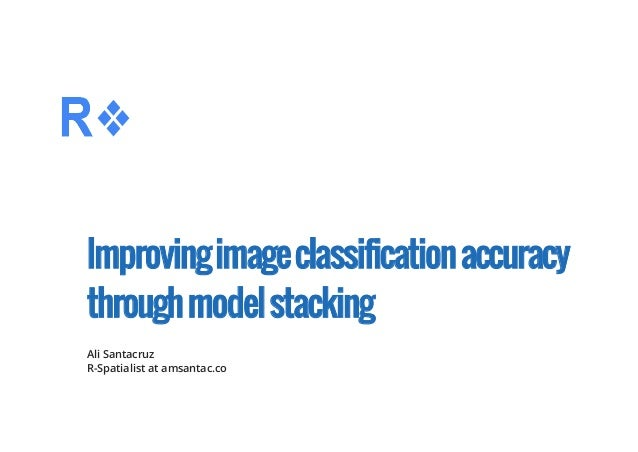Successfully reported this slideshow.
We use your LinkedIn profile and activity data to personalize ads and to show you more relevant ads. You can change your ad preferences anytime.Upcoming SlideShare
Loading in …5
×

# Improving classification accuracy through model stacking

15,339 views

Published on

The video presenting the content for these slides and all the related materials including source code and sample data can be downloaded from this link: http://amsantac.co/blog/en/2016/10/22/model-stacking-classification-r.html.

Model ensembling comprises a set of methods that aims to increase accuracy by combining the predictions of multiple models together.

Ensemble methods can be categorized based on their approach for combining classifiers: one approach is to use similar classifiers and to combine them together using techniques such as bagging, boosting or random forests. A second approach is to combine different classifiers using model stacking.

In this presentation I provide an example of model stacking applied to the classification of a Landsat image.

Published in: Data & Analytics
• Full Name
Comment goes here.

Are you sure you want to Yes No
Your message goes here• Be the first to comment

• Be the first to like this

### Improving classification accuracy through model stacking

1. 1. Improvingimageclassificationaccuracy throughmodelstacking Ali Santacruz R-Spatialist at amsantac.co
2. 2. Keyideas Model ensembling increases accuracy by combining predictions of multiple models together Approaches for model ensembling: The separate predictors must have low correlation to allow the combined predictor to get the best from each model · · Use similar classifiers and combine them together Combine different classifiers using model stacking ­ ­ · 2/14
3. 3. Modelstackingexample:Imageclassification Let's  import  the  image  to  be  classified  (Landsat  7  ETM+,  path  7  row  57,  taken  on  2000­03­16 converted to surface reflectance and provided by USGS) and the shapefile with training data: library(rgdal)  library(raster)  library(caret)    set.seed(123)    img <‐ brick(stack(as.list(list.files("data/", "sr_band", full.names = TRUE))))  names(img) <‐ c(paste0("B", 1:5, coll = ""), "B7")     trainData <‐ shapefile("data/training_15.shp")  responseCol <‐ "class"  3/14
4. 4. Extractdatafromimagebands dfAll = data.frame(matrix(vector(), nrow = 0, ncol = length(names(img)) + 1))     for (i in 1:length(unique(trainData[[responseCol]]))){                              category <‐ unique(trainData[[responseCol]])[i]    categorymap <‐ trainData[trainData[[responseCol]] == category,]    dataSet <‐ extract(img, categorymap)    dataSet <‐ sapply(dataSet, function(x){cbind(x, class = rep(category, nrow(x)))})    df <‐ do.call("rbind", dataSet)    dfAll <‐ rbind(dfAll, df)  }  4/14
5. 5. Createpartitionfortraining,testandvalidation sets # Create validation dataset  inBuild <‐ createDataPartition(y = dfAll\$class, p = 0.7, list = FALSE)  validation <‐ dfAll[‐inBuild,]  buildData <‐ dfAll[inBuild,]    # Create training and testing datasets  inTrain <‐ createDataPartition(y = buildData\$class, p = 0.7, list = FALSE)  training <‐ buildData[inTrain,]  testing <‐ buildData[‐inTrain,]  5/14
6. 6. Balancingadatasetbyundersampling source("undersample_ds.R")  undersample_ds  function (x, classCol, nsamples_class)   {      for (i in 1:length(unique(x[, classCol]))) {          class.i <‐ unique(x[, classCol])[i]          if ((sum(x[, classCol] == class.i) ‐ nsamples_class) !=               0) {              x <‐ x[‐sample(which(x[, classCol] == class.i), sum(x[,                   classCol] == class.i) ‐ nsamples_class), ]          }      }      return(x)  }  6/14
7. 7. Balancetrainingdataset nsamples_class <‐ 600    training_bc <‐ undersample_ds(training, "class", nsamples_class)  7/14
8. 8. Buildseparatemodelsinthetrainingdataand examinetheircorrelation # Random Forests model  set.seed(123)  mod.rf <‐ train(as.factor(class) ~ B3 + B4 + B5, method = "rf", data = training_bc)  pred.rf <‐ predict(mod.rf, testing)  # SVM model  set.seed(123)  mod.svm <‐ train(as.factor(class) ~ B3 + B4 + B5, method = "svmRadial", data = training_bc)  pred.svm <‐ predict(mod.svm, testing)  results <‐ resamples(list(mod1 = mod.rf, mod2 = mod.svm))   modelCor(results)               mod1        mod2  mod1  1.00000000 ‐0.02574656  mod2 ‐0.02574656  1.00000000  8/14
9. 9. Createanewdatasetcombiningthetwo predictors Fit a stacked model to relate the class variable to the two predictions: predDF <‐ data.frame(pred.rf, pred.svm, class = testing\$class)  predDF_bc <‐ undersample_ds(predDF, "class", nsamples_class)  set.seed(123)  combModFit.gbm <‐ train(as.factor(class) ~ ., method = "gbm", data = predDF_bc,                           distribution = "multinomial")  combPred.gbm <‐ predict(combModFit.gbm, predDF)  9/14
10. 10. Overallaccuracybasedontestdataset # RF model accuracy  confusionMatrix(pred.rf, testing\$class)\$overall   Accuracy   0.9812897   # SVM model accuracy  confusionMatrix(pred.svm, testing\$class)\$overall  Accuracy   0.967816   # Stacked model accuracy  confusionMatrix(combPred.gbm, testing\$class)\$overall   Accuracy   0.9838786   10/14
11. 11. Validation pred1V <‐ predict(mod.rf, validation)  pred2V <‐ predict(mod.svm, validation)  predVDF <‐ data.frame(pred.rf = pred1V, pred.svm = pred2V)  combPredV <‐ predict(combModFit.gbm, predVDF)  11/14
12. 12. Overallaccuracybasedonvalidationdataset accuracy <‐ rbind(confusionMatrix(pred1V, validation\$class)\$overall,                     confusionMatrix(pred2V, validation\$class)\$overall,                     confusionMatrix(combPredV, validation\$class)\$overall)  row.names(accuracy) <‐ c("RF", "SVM", "Stack")  accuracy          Accuracy  RF    0.9817141  SVM   0.9658993  Stack 0.9830320  12/14
13. 13. Producer'saccuracybasedonvalidationdataset prod_acc <‐ rbind(confusionMatrix(pred1V, validation\$class)\$byClass[, 1],                    confusionMatrix(pred2V, validation\$class)\$byClass[, 1],                    confusionMatrix(combPredV, validation\$class)\$byClass[, 1])  row.names(prod_acc) <‐ c("RF", "SVM", "Stack")  round(prod_acc, 4)        Class: 1 Class: 2 Class: 3 Class: 5 Class: 6 Class: 7  RF      0.9927   0.9748   0.9769   0.9867        1   0.9982  SVM     0.9913   0.9439   0.9615   0.9905        1   0.9914  Stack   0.9927   0.9752   0.9779   0.9963        1   0.9914  13/14
14. 14. Furtherresources For a detailed explanation please see: Also check out these useful resources: · This post in my blog (includes link for downloading sample data and source code) And this video on my YouTube channel ­ ­ · Kaggle ensembling guide How  to  build  an  ensemble  of  Machine  Learning  algorithms  in  R  (ready  to  use  boosting, bagging and stacking) ­ ­ 14/14